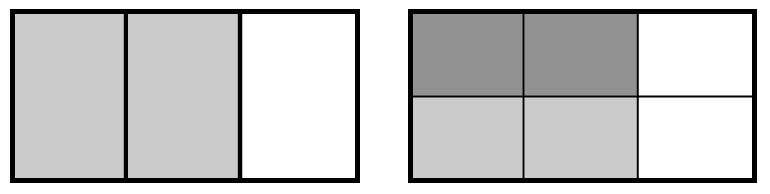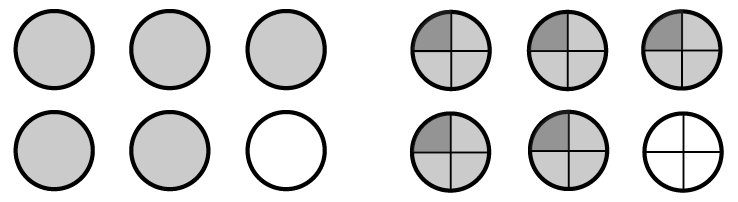### Home > CC1 > Chapter 7 > Lesson 7.3.4 > Problem7-124

7-124.

Each of the following pairs of diagrams shows a first and second step that could be used to represent a multiplication problem. For each pair, write a multiplication problem and its solution.

1.What portion does the first figure represent? The second figure further divides the portion from the first figure.
Into how many pieces does it divide the original portion?

$\left ( \frac{1}{2} \right )\left ( \frac{2}{3} \right )$

What is the solution to this multiplication problem?

2.This problem is very similar to part (a). What portion of the circles are darkened in the first figure?
Into how many pieces are the circles divided in the second figure?